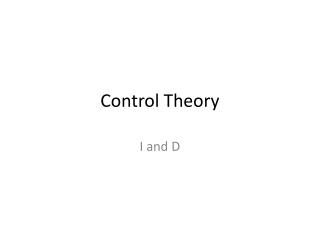DownloadDownload PresentationControl Theory

# Control Theory

Télécharger la présentation## Control Theory

- - - - - - - - - - - - - - - - - - - - - - - - - - - E N D - - - - - - - - - - - - - - - - - - - - - - - - - - -
##### Presentation Transcript

1. ControlTheory I and D

2. The formulas

3. About the pre-class quiz • I could solve it • I couldn’tgetstarted • I could start, butI’mstucksomewhere • I didn’ttry [Default] [MC Any] [MC All]

4. Integral Action When an integrator is present in the loop: steady state error = 0 Therefore one can add an integral in the control action. The PI controller now looks like (in the time domain): TI is the ‘reset time’: it’s a measure for the time over which this integral is ‘smeared out’. A smaller reset time gives a stronger influence over a shorter period of time. (So notice: to put the I action off, the reset time should be infinity!)

5. The TF of the PI controller • Has a zero in zero and no poles • Has a zero in zero and one pole • Has a pole in zero and no zeros • Has a pole in zero and one zerp [Default] [MC Any] [MC All]

6. Integral Action Onecanaddanintegral in the controlaction. The PI controllernowlookslike (in the Laplace domain):

7. First example

8. Example 1: The TF of the closed loop • Is of first order • Is of second order and onepole lies in 0 • Is of second order and contains a zero in 0 • Is of second order and contains a zero • Is of third order [Default] [MC Any] [MC All]

9. First example Calculate the steady state error. Whatabout the steady state error of the controlproblem (Tp,d is not zero)?

10. Secondexample TakeKc=1 Canyoumake the polescriticallydamped? Whatabout the overshoot?

11. Secondexample (Kc= 1)

12. Derivative Action • When there’s too much oscillation, this can sometimes be solved by adding a derivative action. This will take into account how fast the error signal is changing. • In the time domain this leads to: • the PD controller: • and the PID controller:

13. Derivative Action PD in the s domain: PID in the s domain:

14. Problem(s) introduced by D-control? • Control action in servo problem becomes “infinitely” high. • High frequencybehavior/noise is amplified. • None of the above. • Both A and B [Default] [MC Any] [MC All]

15. Bode plots! Bode plot PD: